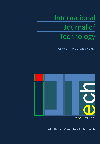### New Modified Left-to-right Radix-R Representation for Integers

Arash Eghdamian, Azman Samsudin

Corresponding email: ae11_com109@student.usm.my

Published at : 29 Apr 2017
IJtech : IJtech Vol 8, No 3 (2017)
DOI : https://doi.org/10.14716/ijtech.v8i3.6570

Cite this article as:
Eghdamian, A., Samsudin, A., 2017. New Modified Left-to-right Radix-R Representation for Integers. International Journal of Technology, Volume 8(3), pp. 519-527
616
Downloads
 Arash Eghdamian School of Computer Sciences, Universiti Sains Malaysia Azman Samsudin School of Computer Sciences, Universiti Sains Malaysia
Email to Corresponding Author

AbstractThis research addresses the problem of finding a minimum Hamming Weight by proposing a left-to-right recoding of integers (from the most significant bit to the less significant one). This representation is the enhanced and modified version of a well-known recoding method called Generalized Non-Adjacent Form (G-NAF). Scanning the digits from the left-to-right is called Modified Generalized Signed Digit Non-Adjacent Form (MGSDNAF), which unlike the G-NAF, presents the ‘nice property’ to be obtained. A ‘nice property’ is one that is based on intuition and is particularly desirable to be obtained in a given context. This processing direction is of great importance because a table of pre-computed values may be used to speed up the scalar multiplication only for that direction. A subsequent advantage is that recoding the exponent in advance is not required. This results in better performances in both running time and memory space. This representation method can reduce the Hamming Weight of integers from about 21.6% for radix 3 to 15.1% for radix 9. These numbers for G-NAF recoding are 16.7% and 8.9% respectively. Comparing these numbers together shows that efficiency of the proposed method in reducing the Hamming Weight is more than the efficiency of G-NAF, which is from 30% (for radix 3) to more than 65% (for radix 9) more efficient in reducing the Hamming Weight. Finally, two radix 3 single scalar multiplication methods for Elliptic Curve Cryptography (ECC), which are based on G-NAF and Left-to-Right MGSDNAF, are compared in order to examine the application of the proposed method in cryptography. The results show that the proposed method can reduce the number of underlying arithmetic operations in single scalar multiplication by 14.1% while G-NAF can only reduce this number by 11.5%.

Cryptography; Elliptic curve; Generalized NAF (G-NAF); Hamming weight; Radix-r representation

Table of Contents